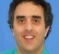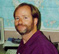## Fourier Transform for a Chirp SignalStarted by 5 years ago7 replieslatest reply 5 years ago2950 views

I was recently asked in a job interview to draw the Fourier Transform of a baseband chirp signal which "chirps" from -40 MHz to +40 MHz over some interval of time T.  Let's assume T is large enough to obtain a reliable analysis of the spectrum, yet finite such that Gibbs phenomenon will have an effect on the spectrum.  Sampling freq is 250 MHz.  Thanks to all.

[ - ]The Fourier Transform of a *single* chirp from f1 to f2 is (in frequency space) a pulse beginning at f1 and running to f2, with a rise portion (and overshoot) at f1 and a decay portion (and overshoot) at f2 (I don't want to call these rise-*time* and fall-*time*, since although the *shape* is the same, but we're dealing with frequencies; not time). If I then repeat the time-domain chirp signal with some period T, then I am really convolving the time-domain chirp signal with an impulse (really, Kronecker delta) train of period T. An impulse train with period T has the Fourier Transform of a impulse train in frequency, with spacing F = 1/T. Well, the Fourier transform of the time-domain *convolution* of two signals is the *product* of the Fourier Transform of each. Voila! The Fourier Transform of the chirp repeating with period T is an impulse train beginning at f1 and ending at f2, with a spacing of F = 1/T. The sampling rate is irrelevant, other than establishing the frequency-granularity with which an FFT will yield frequency component values.

[ - ]The spectrogram is pretty easy to draw, i.e., a line in the time-frequency plane that shows the peak energy moving across the frequencies that the chirp occupies.  From that you can slice the time window of the example FT, and if it includes the entire chirp, then the ideal FT of the ideal chirp is a rectangular function that starts at the starting frequency and ends and the final frequency.

So, in your example case the ideal FT would be a rectangular function from -40MHz to +40MHz, like the ideal LPF with the same cutoff.   If the system is sampled at 250MHz it is more than adequately sampled, so no need to worry about aliasing.

If it was me I'd draw the ideal case and then just talk about how a finite window would smooth the edges, make it ring, etc., etc.

So what did you say?

[ - ]Thank you both for your replies. I will answer Slartibartfast first, if I may.

I came to the same conclusion that the FT of the chirp will be rectangular in the freq domain, as you indicate, in which the peak energy will be uniform over the chirp frequencies. What intrigued me were two issues.  1: This spectrum will be the same as a time domain sinc pulse (ideal LPF as you mentioned). Are we intuitively comfortable with the fact that 2 completely different signals will have identical spectrums?

2: Being that it is a chirp pulse of length T, we are effectively multiplying by a rectangular pulse of length T in the time domain, yielding a convolution in the freq domain between our chirp’s spectrum (rectangular) and a sinc pulse due to the rectangular time-domain window. What we should arrive at, is a spectrum quite similar to that of a finite-in-length LPF, which will be rippled due to Gibbs Phenomenon.

Now, as achesir mentioned, there will be overshooting in the rise and decay portions of the spectrum. Is this the “ripple” we are expecting?

BTW, I was on the right track in the interview, but had the above questions which were left for "homework".

[ - ]Regarding "Are we intuitively comfortable with the fact that 2 completely different signals will have identical spectrums?"

They have identical magnitudes, but different phase. The sinc pulse has linear phase, but the chirp has parabolic phase. Parabolic phase means that the frequency components are delayed different amounts in the time domain. Hence the time domain sweep from f1 to f2.

[ - ]Thank you for pointing out this oversight on my part.  I was just now shown of a paper that deals with the analytics of linear sweeps:

Mathematics of Linear Sweeps, by David Aldridge, Canadian Journal of Exploration Physics, June 1992.

I assume that he will show how to derive the parabolic phase response you mention.

[ - ]